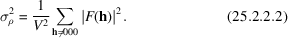International
Tables for
Crystallography
Volume F
Crystallography of biological macromolecules
Edited by M. G. Rossmann and E. Arnold

International Tables for Crystallography (2006). Vol. F, ch. 25.2, p. 709   | 1 | 2 |

## Section 25.2.2.5.1. Scaling

K. D. Cowtan,b* K. Y. J. Zhangc and P. Maind

#### 25.2.2.5.1. Scaling

| top | pdf |

All forms of map modification are affected by the overall temperature factor of the data, and histogram matching in particular is critically dependent on the accurate determination of the scale factor. Wilson statistics have been found inadequate for scaling in this case, especially when the data resolution is worse than 3 Å, because of the dip in scattering below 5 Å.

More accurate estimates of the scale and temperature factors may be achieved by fitting the data to a semi-empirical scattering curve (Cowtan & Main, 1998). This curve is prepared using Parseval's theorem, which relates the sum of the intensities to the variance of the map:Thus, the sum of the intensities in a particular resolution shell is proportional to the difference in variance of maps calculated with and without that shell of data. The empirical curve is therefore calculated from the variance in the protein regions of a group of known structures, calculated as a function of resolution. The curve is scaled to the protein volume of the current structure, and a correction is made for the solvent, which is assumed to be flat.

The overall temperature factor is removed, and an absolute scale is imposed by fitting the data to this curve. The use of sharpened F's (with no overall temperature factor) is necessary for histogram matching and often increases the power of averaging for phase extension.

Since the solvent content is used in scaling the data, it is important that this value be entered correctly. However, the volume of the solvent mask may be varied independently of the true solvent content, as discussed in Section 25.2.2.3.

### References

Cowtan, K. D. & Main, P. (1998). Miscellaneous algorithms for density modification. Acta Cryst. D54, 487–493.Google Scholar